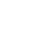# 单层钢结构厂房之钢架设计（一）截面设计

B=200mm，H=450mm，tw=8.0mm，tf=12.0mm，A=2052.5px2

Ix=704525px4，Wx=31300px3，ix=463.25px

Iy=40050px4，Wx=4004.9999999999995px3，ix=110.5px

（二）构件验算1．构件宽厚比的验算

2．刚架梁的验算

(1)抗剪验算

fv=125N/mm2

Vu=hwtwfv=426×8×125=426000N=426.0KN

Vmax=77.60KN

(2)弯、剪、压共同作用下的验算

N=39.89KN，V=77.60KN，M=193.30KN·N

=220.90KN·m>M=193.30KN·m，取M=Mf

(3)整体稳定验算

N=39.89KN，M=193.30KN·m

A．梁平面内的整体稳定性验算。

λx=lx/ix=18090/185.3=97.63<[λ]=150，b类截面，查表得ψx=0.570=165.15N/mm2

B．横梁平面外的整体稳定验算

λy=μsl/iy0=3015/44.2=68.2，b类截面，查表得ψy=0.762(4)按《钢结构设计规范》(GB50017－2003)校核横梁腹板容许高厚比(5)验算檩条集中荷载下的局部受压承载力

F=(1.2×0.27×6 1.4×3.00)×3=18.43KN

Lz=a 5hy 2hR=70 5×12 0=130mm=130.65 N/mm2<1.2f=258 N/mm2，满足要求。

3．刚架柱的验算

fv=125N/mm2

Vu=hwtwfv=426×8×125=426000N=426.0KN

Vmax=32.21KN

(2)弯、剪、压共同作用下的验算

N=81.22KN，V=32.21KN，M=193.30KN·N(3)整体稳定验算

A．刚架柱平面内的整体稳定性验算。

K2/K1=0.332，查表得柱的计算长度系数μ=2.934。

λx=lx/ix=17604/185.3=95。0<[λ]=150，b类截面，查表得ψx=0.588=181.45N/mm2

B．刚架柱平面外的整体稳定验算

λy=μsl/iy0=3000/44.2=67.9，b类截面，查表得ψy=0.764(4)按《钢结构设计规范》(GB50017－2003)校核刚架柱腹板容许高厚比4．验算刚架在风荷载作用下的侧移μ

Ic=Ib=704525px4，ζt= Icl/hIb=18000/6000=3.0

H=0.67W=0.67×13.56=9.09KN

（三）节点验算1．梁柱连接节点

(1) 螺栓强度验算(2)端板厚度验算2．横梁跨中节点(2)端板厚度验算3． 柱脚设计

(1)柱脚内力设计值Nmax=102.82KN，相应的V=32.21KN；

Nmin=19.05KN，相应的V=4.30KN。

(2)由于柱底剪力较小，

Ｖmax=32.21KN<0.4Nmax=41.13KN，故一般跨间不需剪力键；但经计算在设置柱间支撑的开间必须设置剪力键。另Nmin>0，考虑柱间支撑竖向上拔力后，锚栓仍不承受拉力，故仅考虑柱在安装过程中的稳定，按构造要求设置锚栓即可，采用4M24。

• 0人已收藏

• 0人已打赏

免费
• 人已点赞

• 分享

+1经验值
+1土木币46.14 万条内容 · 839 人订阅